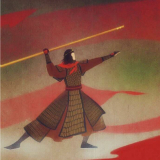### qqq★晒月光★Visual C++中error spawning cl.exe能帮看下这个错怎么改吗？网上的方法都试过了（崩溃 ）sake.#include <stdio.h> #include <stdlib.h> #include <time.h> main() { int t, i, a, b; time(&t); srand(t); a = rand()%100; b = rand()%100; printf("a=%d\nb=%d\n",a,b); printf("a+b=%d\n",a+b); } 老师这程序哪里错了呀if语句里的条件真假，都会执行a ？没有else？ { if(a%2!=0&&(ch>='a'&&ch<='z')) ch=ch-'a'+'A'; a++; putchar(ch); } 如果 if(a%2!=0&&(ch>='a'&&ch<='z')是真，为什么a++还是执行的，不是else才执行吗？#include <stdio.h> main() { char a=0,ch; while((ch=getchar())!='\n') { if(a%2!=0&&(ch>='a'&&ch<='z')) ch=ch-'a'+'A'; a++; putchar(ch); } printf("\n"); }QF{int i,n; char *p ; p=ss; n=strlen(ss); for(i=0;i<n;i++) if(i%2==1&&*p>='a'&&*p<='z') {ss[i]=*(p+1)-32; p+=2;}为什莫不能把字符串下标为奇数位的按大写输出来那？字母可以输出来，但是数字不能原样输出为什莫？